# Crafting web apps using finite state machines - Part 1

15 Nov 2019## Dan Bunea

See author's bio and posts

# Crafting web apps using finite state machines - Part 1

Code on Gitlab

• PROBLEM
• How should we start crafting a web app?
• SOLUTION
• THEORY
• Strategy
• Goals
• Tactics
• Patterns
• Techniques
• PRACTICE
• Step 1 Draw the diagram of the UI state machine
• Step 2: Domain modelling
• Step 3: Solve the data problem
• Step 4: Build the UI
• Goals revised
• Part II - what will be discussed

# PROBLEM: How should we start crafting a web app?

The requirements come, we have some UI mockups and now we need to start developing a web app. What is the first step we need to do?

Let's go back a bit. If this was not a specific web app but rather a generic one, then:

1. What would our strategy be like?
2. What would be our goals?
3. Which steps would we have to do?
4. Which patterns could help us?
5. What tactics could we use?

In the following lines I will try to address exactly this questions. We'll start with a little bit of theory, then we'll put it in practice:

# SOLUTION

## Strategy

We'll have to think a bit strategically and a little bit tactically. For the strategy, what would be our goals:

### Goals

• Break the complexity down (state machines, separation of concerns)
• Build robustness into the system (atomicity with rollback)

Ok, but how can this be done?

Well, we'll do it in 4 Steps:

1. Finite state machine diagram (state/transitions starting from the UI)
2. States (domain modelling for the states)
3. Transitions (TDD the controller)
4. Presentation/UI for the states (TDD the view)

Steps don't need to be sequential, in fact it is recommended to do the last two in parallel.

## Tactics

### Patterns

We will be using a few patterns:

• finite state machines
• separation of concerns using MVC
• atomicity = all or nothing

### Techniques

and a few Techniques:

• TDD - for the non UI
• TDD - for the UI
• Design by contract

## PRACTICE

We'll rebuild the famous TodoMVC web application (even though some people suffer from TodoMVC fatigue), focusing on what we said before.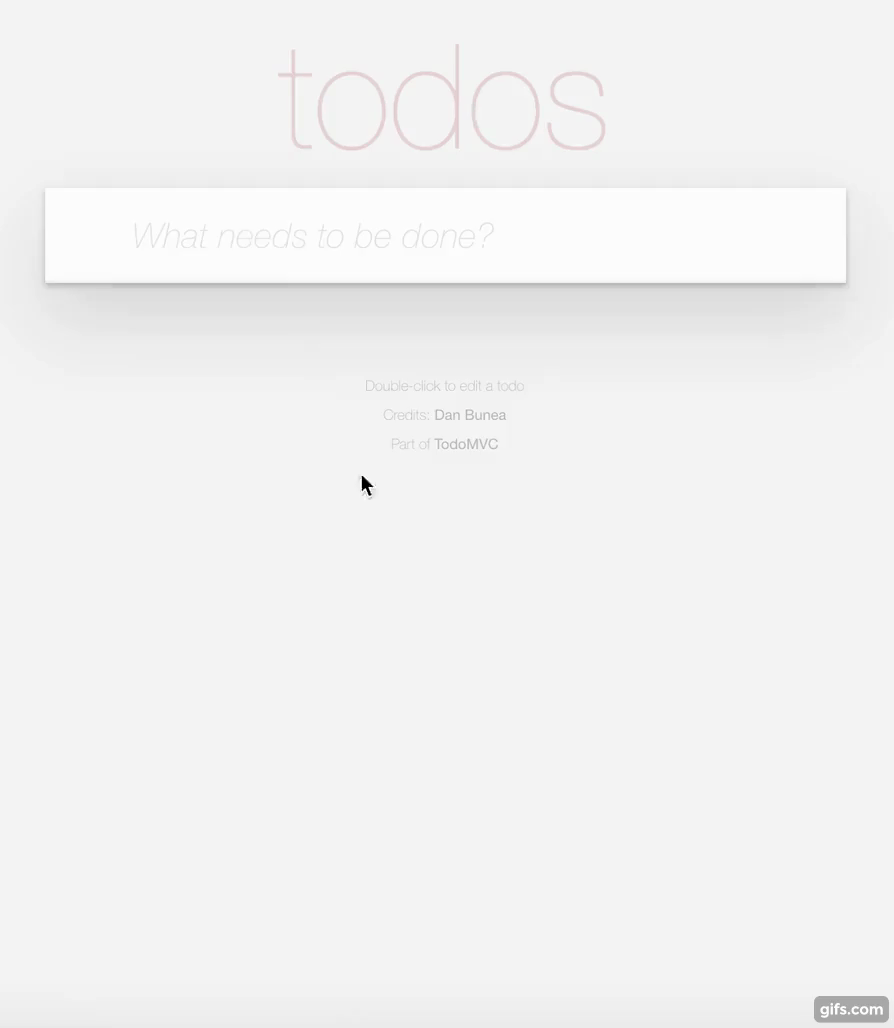### Step 1 Draw the diagram of the UI state machine

Our purpose is to create a state diagram with all the states and transitions we think we'll need. This is not an easy step, but it can clarify the entire development process further on. Let's see what can be done.

Actions:

• list
• filter
• check/unckeck
• edit
• delete

From the screens it also look like we'll have the following States: list (filtered or not)

• edit

and now let's put it in a diagram: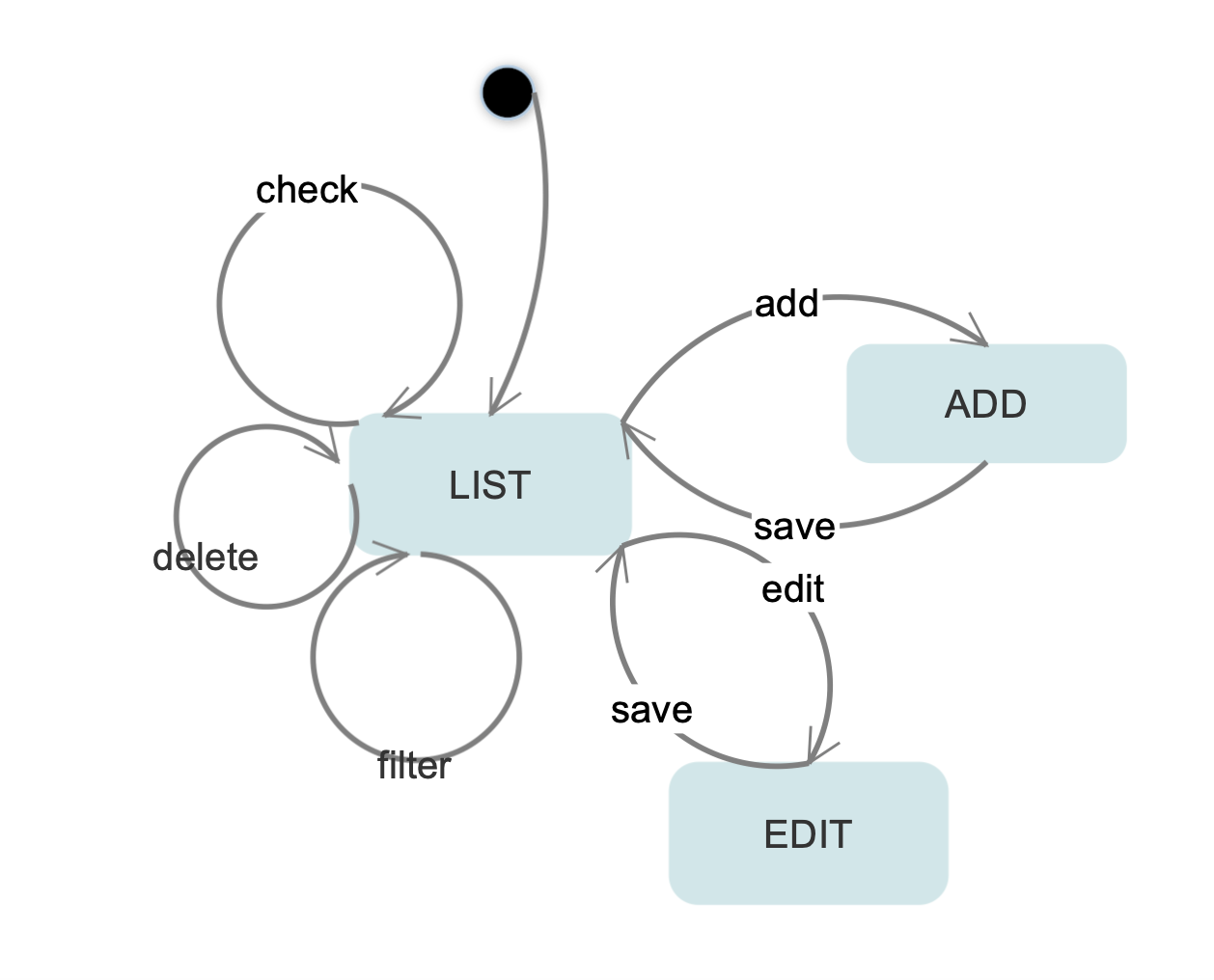Now using this diagram we'll move to step 2 and we'll do the code according to it.

### Step 2: Domain modelling

We will separate the different concerns using the MVC pattern. All the logic will be in the controller, the data in the model and we'll add the presentation later (or someone else could do it in parallel).

Our data will have to be able to represent all the states in the diagram above: list/filter, add and edit

``````;What is a todo?

{:text "todo", :done? true}

;How do we know the status?
;When do we filter?

{:context {:status "list"
:filter true}
:todos [{:text "todo 1" :done? true}
{:text "todo 2" :done? false}]}

;How do we know which do we edit?
;How do we order the todos?

{:context {:status "edit"
:filter true
:selected-id 1},
:todos {
:1 {:id 1, :text "todo 1", :done? true, :time 1}
:2 {:id 2, :text "todo 2", :done? false, :time 2}
}}

;Let's carve it in stone: (require '[clojure.spec.alpha :as s])

;SPEC
(s/def ::filter #(or (false? %) (true? %)))
(s/def ::filter-with-nil #(or (nil? %) (false? %) (true? %)))
(s/def ::selected-id nat-int?)
(s/def ::context (s/keys :req-un [::status] :opt-un [::filter-id ::selected-id]))

(s/def ::id nat-int?)
(s/def ::text string?)
(s/def ::done? boolean?)
(s/def ::time number?)
(s/def ::todo (s/keys :req-un [::id ::text ::done? ::time]))
(s/def ::todos (s/map-of keyword? ::todo))

(defn edit-mode-has-a-valid-selected-id? [state]
(if (= "edit" (get-in state [:context :status]))
(some (into #{} (map :id (vals (:todos state)))) [(get-in state [:context :selected-id])])
true
))

(s/def ::model (s/and (s/keys :req-un [::context] :opt-un [::todos])
edit-mode-has-a-valid-selected-id?
))

(comment
(s/valid? ::model {:context {:status "edit"
:filter true
:selected-id 1}
:todos {
:1 {:id 1, :text "todo 1", :done? true, :time 1}
:2 {:id 2, :text "todo 2", :done? false, :time 2}
}}))
``````

### Step 3: Solve the data problem

Now we know how we could represent our states as data in the model, let's test drive the different transitions which will al be functions in the controller. Normally I start with a plan, where I will know what I want to test.

The plan of the tests:

• [ ] controller-should
• [ ] initialize-in-list-mode
• [ ] check&uncheck
• [ ] delete
• [ ] toggle-filters
• [ ] set a filter
• [ ] remove all filters
• [ ] save-a-new-todo
• [ ] set-list-mode
• [ ] set-one-for-edit
• [ ] save-a-changed-todo

Then we'll write the first one:

``````(deftest initialize-in-list-mode
(is (= {:context {:status "list"} :todos   {}}
(init!))))
``````

which will obviously

Test fails! Good... now we write the code to make it pass:

``````;THE MODEL
(def model (atom {:context {:status "list"}}))

;THE CONTROLLER
(defn commit! [value atom-to-change]
(reset! atom-to-change value))

(defn init! []
(-> @model
(assoc :todos {})
(assoc-in [:context :status] "list")
(commit! model)))

;THE TEST
(deftest initialize-in-list-mode
(is (= {:context {:status "list"} :todos {}}
(init!))))
``````

Any refactorings? No, let's move to the second test and so on until we solve the entire data problem, one test at a time, making sure it fails, then making it pass, then refactoring the code. We end up with this: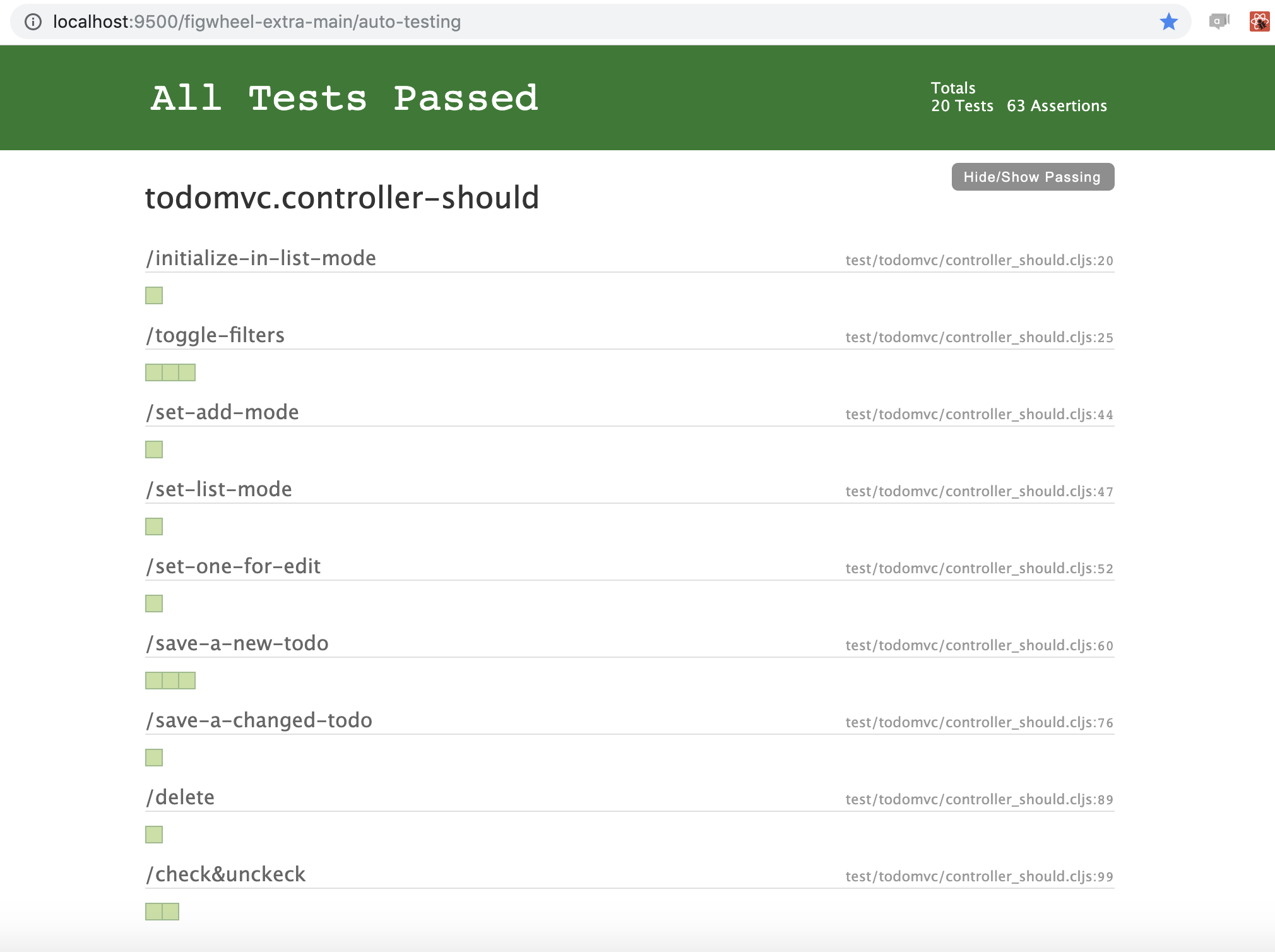Solve the data problem (TDD the model/controller) Done!

The code is merged here: https://gitlab.com/danbunea/how-to-solve-it-crafting-web-apps/merge_requests/1/diffs

Code:

Now let's have another look again to see the correspondence between the transitions and the controller functions:while the data in the model corresponds to: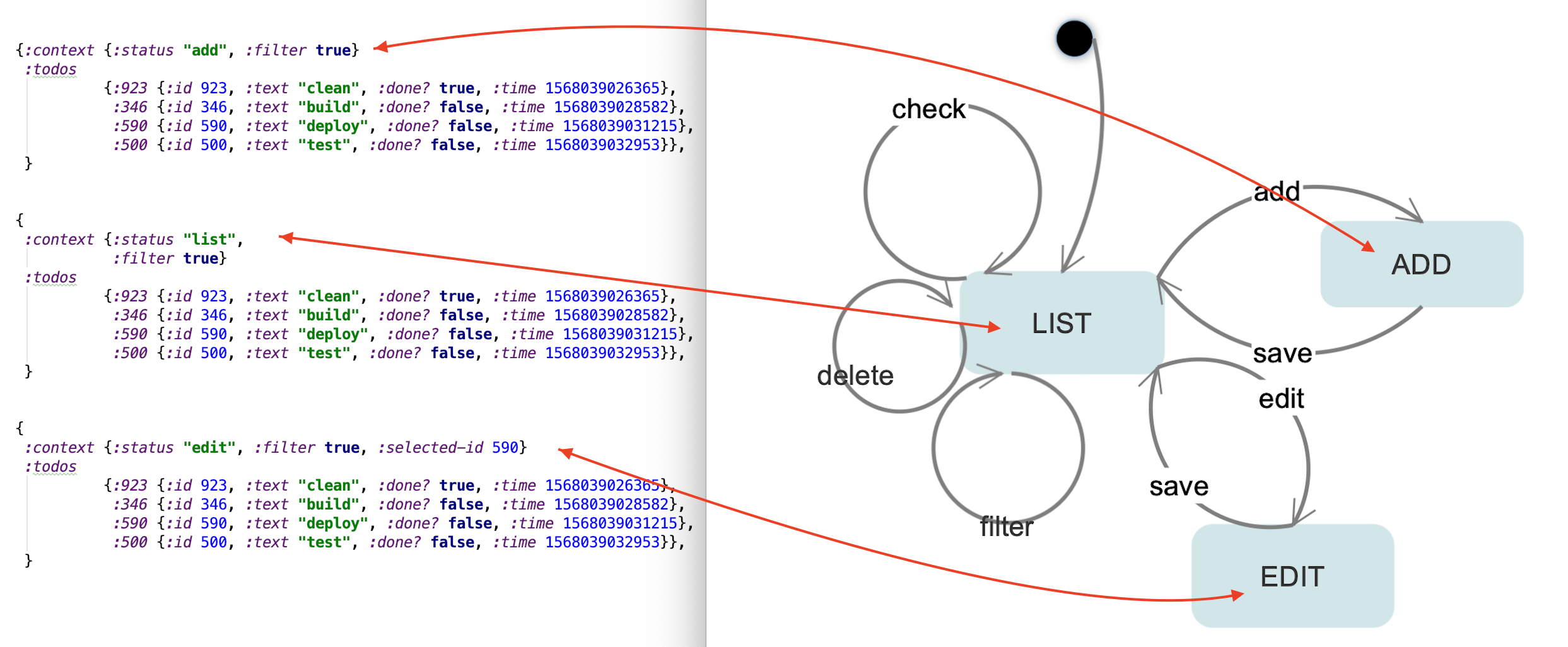It is also worth mentioning that we're using the Reagent library, where the model is an atom that is watched for changes. When the atom is changed (in our case by the controller functions, thus the transitions) it will tell the UI to repaint.

### Step 4: Build the UI

We will need now to make sure that our states can be represented on the screen.

For instance when we're in list mode, filtered: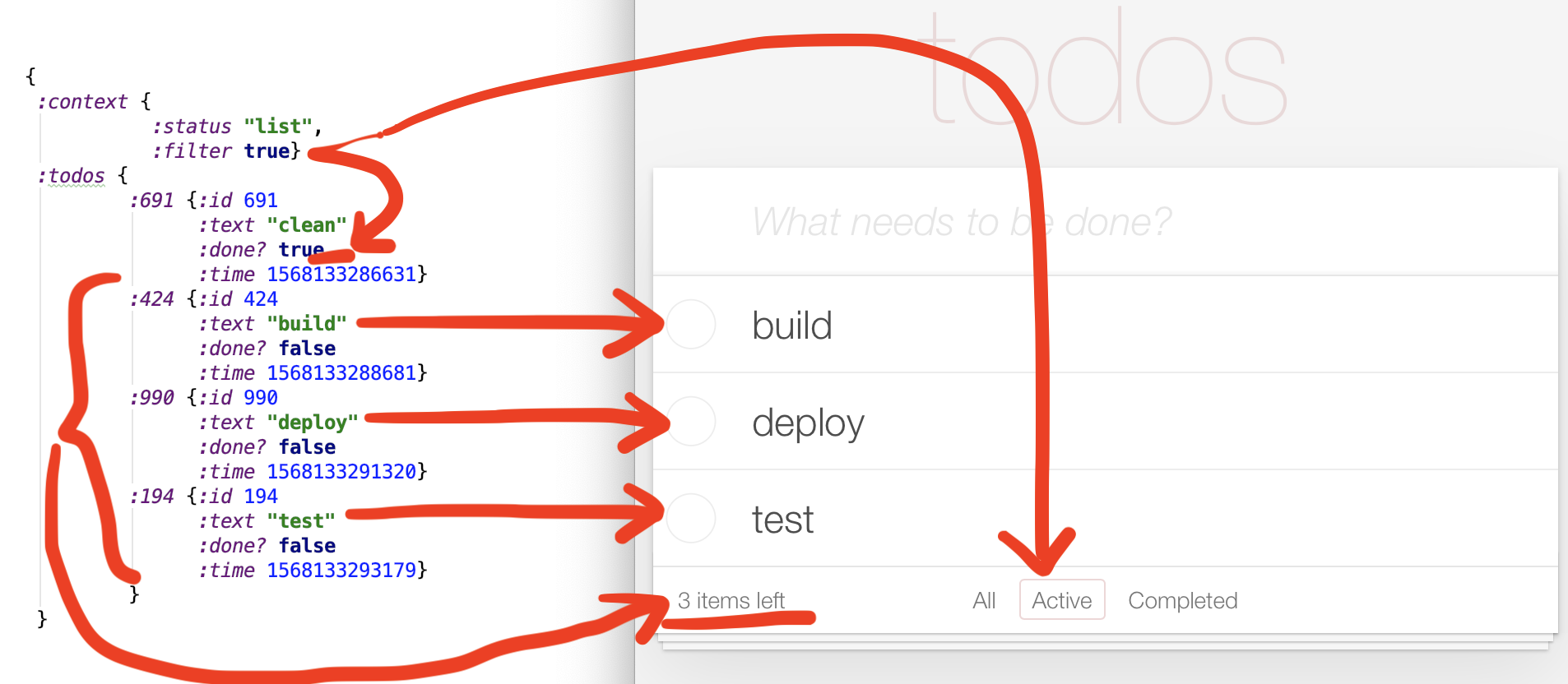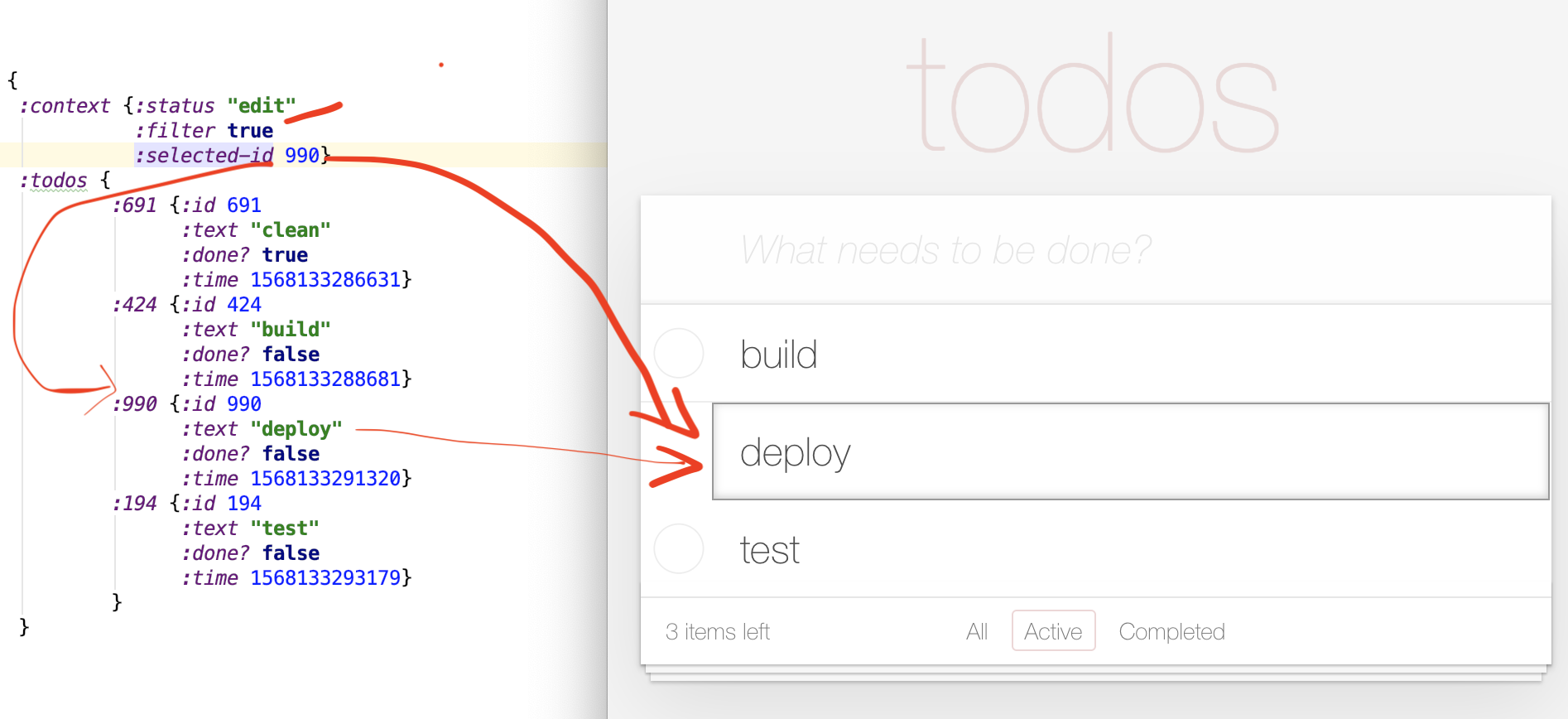Now that we know how the data corresponds to the UI, we can move on to doing the actual UI. We'll use react/reagent to break the UI into components:

``````screen_component
todo_input_component
text_input_component
todos_list_component
todo_list_item
todos_count_component
todos_filter_component
``````

The final version will be like: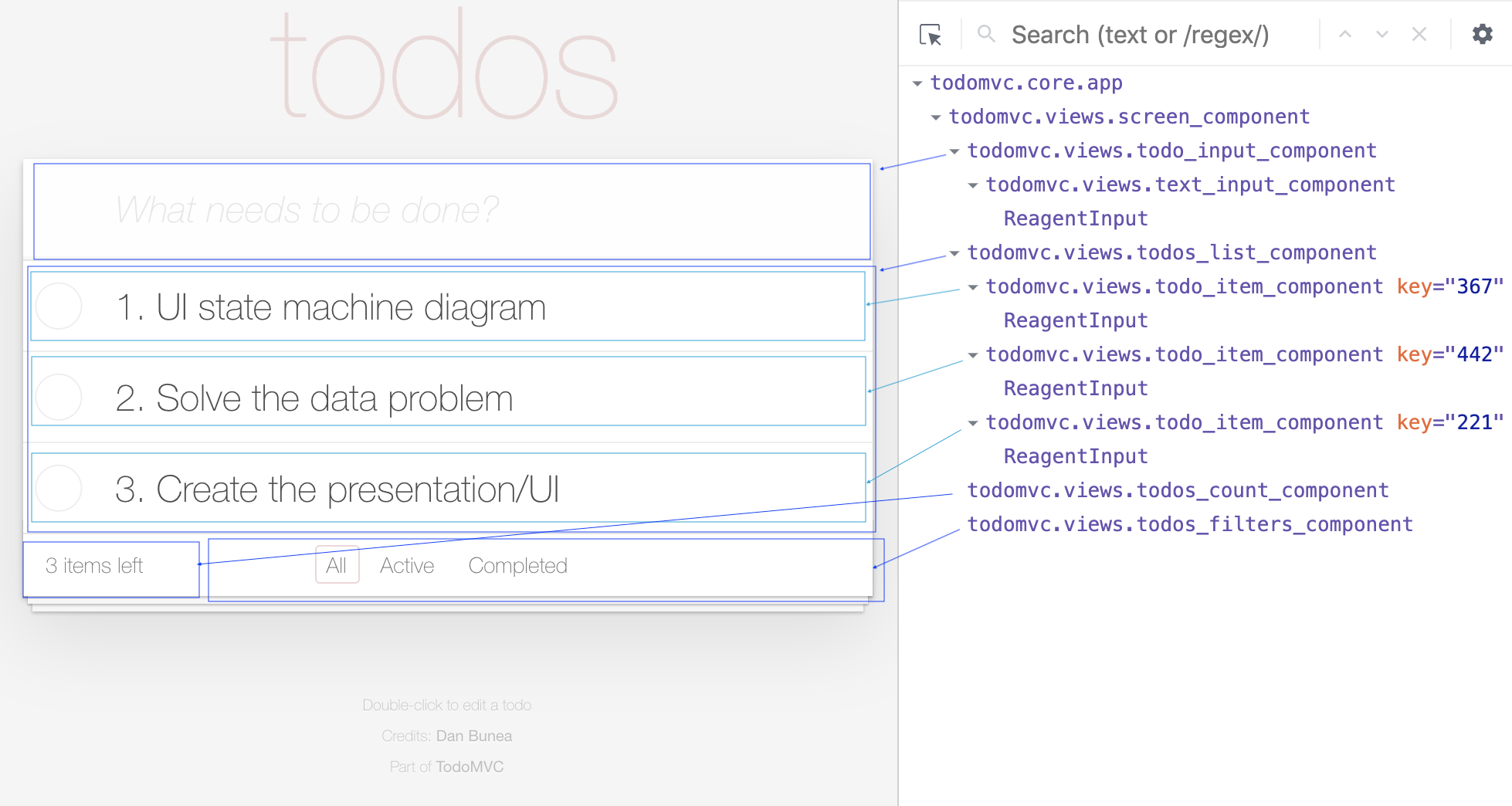Then we'll test drive the entire UI starting by planning the tests:

• [ ] views_should
• [ ]  render-the-main-screen
• [ ]   render all sections
• [ ]  not render main section when no todos
• [ ]  render-input-text-component
• [ ]  use-keys-on-input-text-component
• [ ]   write something and hit enter
• [ ]   hitting enter with no text
• [ ]   hitting esc
• [ ]  render todo input component
• [ ]  render-todos-list-component
• [ ]   no filter
• [ ]   filter active
• [ ]   filter completed
• [ ]  render-todo-item-component
• [ ]   normal mode
• [ ]   completed mode
• [ ]   editing mode
• [ ]  toggle a todo item component
• [ ]  render-todos-count-component
• [ ]   no item left
• [ ]   1 item left
• [ ]   2 items left
• [ ]  render-todos-filters-component
• [ ]   render no filter
• [ ]   render filter active
• [ ]   render filter completed
• [ ]  invoke-controller-filter-when-clicking-filters-in-todos-filters-component

It is worth mentioning that TDD-ing the UI can be split into two types of tests: - render tests and - interaction tests

For the render tests you send some data to your components, render it then check how it's rendered. We start with a render test (we'll use enzyme):

``````(deftest render-the-main-screen
(testing "render all sections"
(let [component [views/screen-component data/one-todo-list-mode-no-filter]
mounted (->> (r/as-element component)
(.mount js/enzyme))]
(is (= 1 (-> mounted (.find ".header") .-length)))
(is (= 1 (-> mounted (.find ".main") .-length)))
(is (= 1 (-> mounted (.find ".footer") .-length)))
(is (= 1 (-> mounted (.find "li.todo") .-length)))
(is (= "0 items left" (-> mounted (.find "strong") .getDOMNode .-innerText)))
)))
``````

the test fails, so then we start writing our first component, making it pass, refactoring etc.

For the interaction tests, you render some data, then click a button and make sure the mocked function that should be invoked is actually invoked:

``````(deftest invoke-controller-filter-when-clicking-filters-in-todos-filters-component
(let [invocations (atom [])
component [views/todos-filters-component nil]
mounted (->> (r/as-element component)
(.mount js/enzyme))]
(with-redefs [controller/filter! #(swap! invocations conj [%])]
(testing "unfilter"
(reset! invocations [])
(-> mounted
(.find "#all")
(.simulate "click"))
(is (= [[nil]] @invocations)))
)))
``````

The code is merged here: https://gitlab.com/danbunea/how-to-solve-it-crafting-web-apps/merge_requests/2/diffs

Code: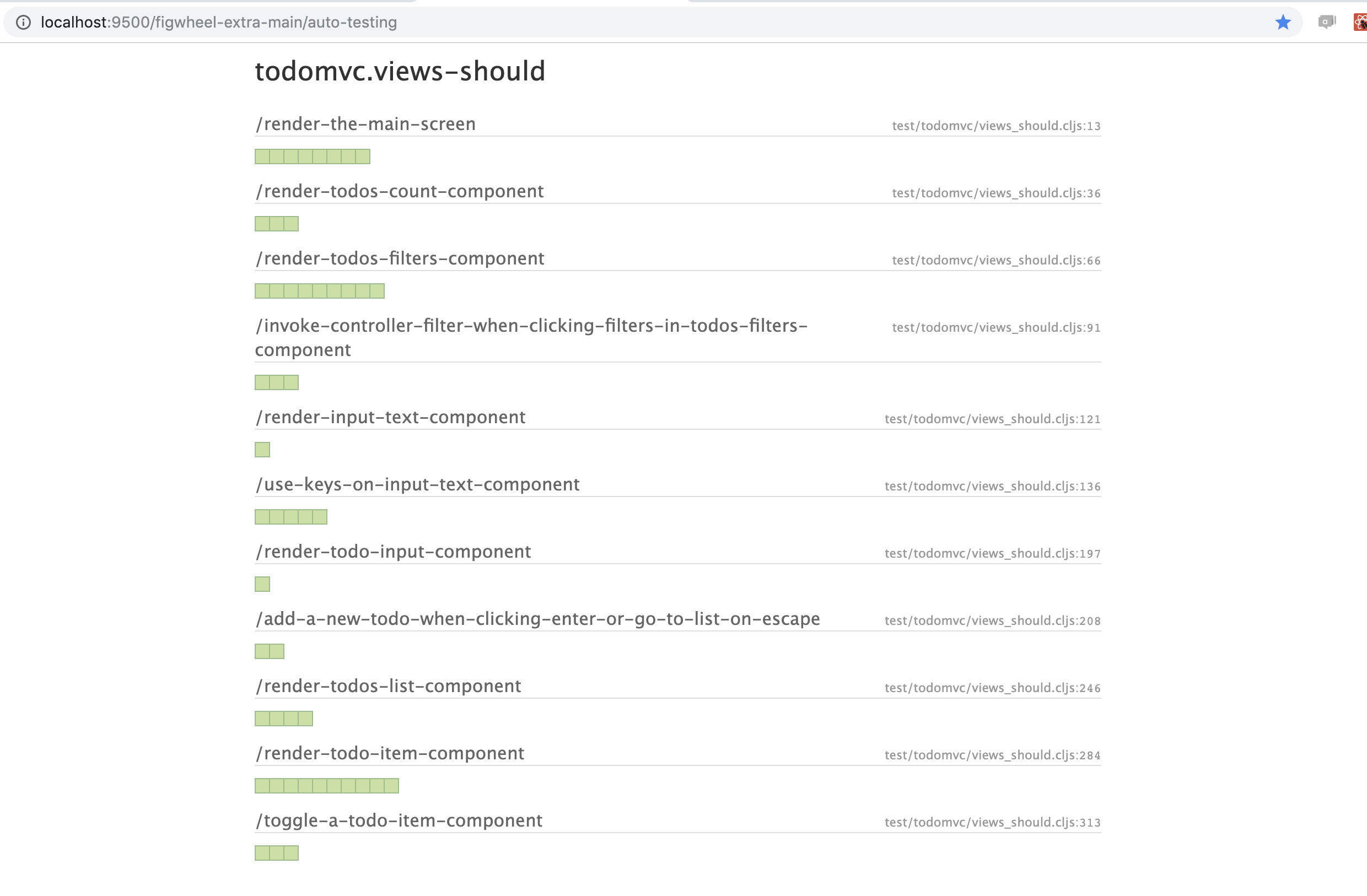## Goals revised

The complexity problem -solved by making a state machine and using separation of concerns.

## Parth II - what remains to be discussed

The Robustness problem - TDD is a clear step forward but we can go further:

• add atomicity into the transitions/operations (all or nothing)
• design by contract, always making sure the states are valid states

But that will follow in part II.